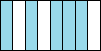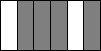# Introduction Of Fractions Online Quiz

#### Complete Python Prime Pack

9 Courses     2 eBooks

#### Artificial Intelligence & Machine Learning Prime Pack

6 Courses     1 eBooks

#### Java Prime Pack

9 Courses     2 eBooks

Following quiz provides Multiple Choice Questions (MCQs) related to Introduction Of Fractions. You will have to read all the given answers and click over the correct answer. If you are not sure about the answer then you can check the answer using Show Answer button. You can use Next Quiz button to check new set of questions in the quiz.Q 1 - The circle below is cut in 6 equal slices. What fraction of the circle is shaded?A - 3/6

B - 5/6

C - 4/6

D - 2/6

### Explanation

Step 1:

The given circle is cut in 6 equal slices.

Step 2:

3 slices are shaded. So the fraction of the circle that is shaded is 3/6.

Q 2 - The circle below is cut in 8 equal slices. What fraction of the circle is shaded?A - 4/8

B - 5/8

C - 3/8

D - 6/8

### Explanation

Step 1:

The given circle is cut in 8 equal slices.

Step 2:

6 slices are shaded. So the fraction of the circle that is shaded is 6/8.

Q 3 - The circle below is cut in 3 equal slices. What fraction of the circle is shaded?A - 3/1

B - 2/3

C - 1/3

D - 3/3

### Explanation

Step 1:

The given circle is cut in 3 equal slices.

Step 2:

1 slice is shaded. So the fraction of the circle that is shaded is 1/3.

Q 4 - The figure below is cut into 8 equal slices. What fraction of the figure is shaded?A - 3/8

B - 5/8

C - 4/8

D - 6/8

### Explanation

Step 1:

The given figure is cut in 8 equal slices.

Step 2:

5 slices are shaded. So the fraction of the figure that is shaded is 5/8.

Q 5 - The figure below is cut into 6 equal slices. What fraction of the figure is shaded?A - 3/6

B - 4/6

C - 5/6

D - 4/8

### Explanation

Step 1:

The given figure is cut in 6 equal slices.

Step 2:

4 slices are shaded. So the fraction of the figure that is shaded is 4/6.

Q 6 - The circle below is cut in 4 equal slices. What fraction of the circle is shaded?A - 1/4

B - 2/4

C - 3/4

D - 4/3

### Explanation

Step 1:

The given circle is cut in 4 equal slices.

Step 2:

3 slices are shaded. So the fraction of the circle that is shaded is 3/4.

Q 7 - The strip below is cut in 4 equal parts. What fraction of the strip is shaded?A - 4/4

B - 3/4

C - 2/4

D - 1/4

### Explanation

Step 1:

The given figure is cut in 4 equal slices.

Step 2:

All 4 slices are shaded. So the fraction of the figure that is shaded is 4/4.

Q 8 - The circle below is cut in 3 equal slices. What fraction of the circle is shaded?A - 3/2

B - 1/3

C - 3/3

D - 2/3

### Explanation

Step 1:

The given circle is cut in 3 equal slices.

Step 2:

2 slices are shaded. So the fraction of the circle that is shaded is 2/3.

Q 9 - The circle below is cut in 6 equal slices. What fraction of the circle is shaded?A - 5/6

B - 4/6

C - 3/6

D - 2/6

### Explanation

Step 1:

The given circle is cut in 6 equal slices.

Step 2:

5 slices are shaded. So the fraction of the circle that is shaded is 5/6.

Q 10 - The circle below is cut in 8 equal slices. What fraction of the circle is shaded?A - 2/8

B - 5/8

C - 6/8

D - 7/8

### Explanation

Step 1:

The given circle is cut in 8 equal slices.

Step 2:

6 slices are shaded. So the fraction of the circle that is shaded is 6/8.

introduction.htm